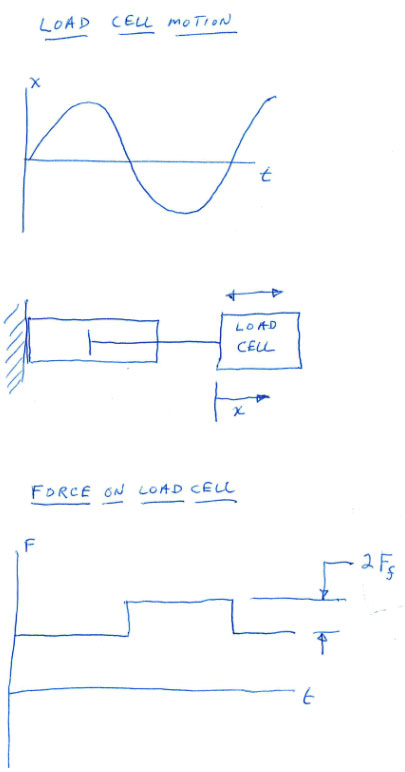# How Airpot Measures Friction in their Airpel Plus Air Cylinders

We at Airpot make three different low friction air cylinder lines under the Airpel name. Many customers have asked us how we measure the friction in these cylinders. Today, we’re going to explain how friction is measured in our Airpel Plus air cylinders.

The Airpel (or standard Airpel) is a low friction air cylinder available with many options, but is actually the highest friction cylinder of the three types. The friction specified is “typical” and each unit is not measured for friction under load.

The Airpel-AB is the lowest friction air cylinder we make. The air bearing piston is actually frictionless but there is non-zero friction in the ball joints that connect the rod to the piston and the rod to the load, so there is a tiny amount of “apparent friction” during the movement because of the small motions of the ball joints. Measuring that the piston is frictionless is not an easy task, but we do it for 100 percent of production units. The actual technique used to measure the friction of an Airpel-AB is a trade secret, but be assured that all units are tested before we ship them.

The Airpel Plus is specified with a friction level of 0.5% MAXIMUM of the load. Before we get into how this friction is measured, let’s discuss this specification. Take a case where the Airpel Plus cylinder is pushing against something with an 80 N nominal force. This means that the air pressure multiplied by the piston area equals 80 N (check your units!).

Friction acts in a direction that opposes the direction of motion. This means that when the rod is pushing against the load at the same time as the rod is slowly extending, the friction force acts in the opposite direction of motion or toward the body of the air cylinder. This means that the actual force applied (F) would be F = 80 N - Ff where Ff is the piston friction. In the same example, but when the rod is slowly retracting, the piston friction force adds to the nominal force, so F = 80 N+Ff

Staying with the Airpel Plus where the friction specification is 0.5% of the load maximum, we can calculate the max friction Ff = 0.5% x 80 N. 0.5% is just 0.005 so Ff = 0.005 x 80 N = 0.4 N. To make sure every unit meets this specification, we supply the Airpel Plus with a very well controlled known pressure. The rod on this low friction air cylinder pushes up against a load cell. A mechanism moves the load cell (and the air cylinder rod) back and force with sinusoidal motion. The amplitude and frequency of the motion are chosen so that accelerations are very small making inertial effects on the load cell insignificant. This change in the direction of the velocity causes the friction force to alternately add and subtract from the nominal force. A purely frictionless air cylinder will not change its force because of this motion, so the load cell output will remain constant. However, a cylinder with friction will cause the load cell to output a square wave, with the top of the wave showing a load of F + Ff and the bottom showing F - Ff. Once we measure the peak-to-peak force value of this square wave we simply calculate Ff = Fpeak-to-peak/2.Feb. 52021
One of the ways our air cylinders have such low friction is that the Airpot (and Airpel) pistons are fit to the cylinder with clearance and therefore, there are no true seals. However, this means that whenever there is a...

### This 3D file is not available.

#### However if you submit this form, our engineers will create the 3D file for you.

Please answer the math question below to prove you are a human!